# C语言_干翻操作符_含义+结合性+优先级+例子分析

hackernew

0收藏

1.+操作符

2.-操作符

（注意与负号运算符区分即可不再赘述）

1，<<操作符

``````#include <stdio.h>
int main()
{
int a = -1;
a = a << 1;
printf("%d",a);
return 0;
}
``````

-----------------------------------------------------------------

eg（都是32位）

10000000000000000000000000000001表示-1

00000000000000000000000000000001表示1

eg:
1 1 1 1 1…1 1(32位)（符号位1说明是负数）补码

1 1 1 1 1…1 0(32位)（补码-1到反码）

1 0 0 0 0…0 1(32位)（符号位不变其余位按位取反到原码）

-----------------------------------------------------------------
a的二进制值在内存中为32个1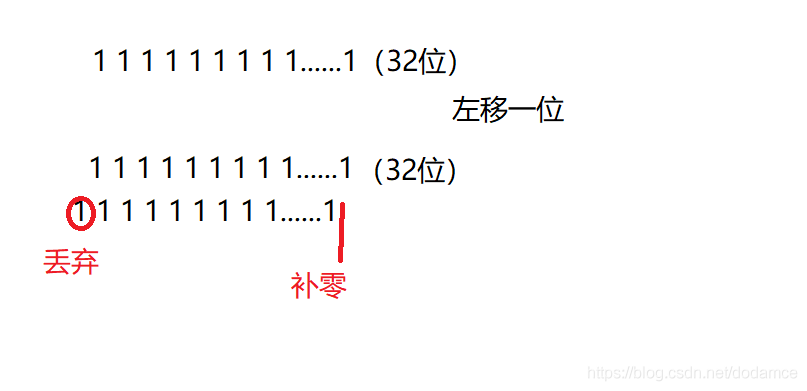2.>>操作符

1，算数右移
2，逻辑右移

vs2019使用的是算数右移

eg:
a=a>>-1(这种写法编译器不支持)

1.==操作符

==判断是否相同时不能判断字符串与字符串相同

2，！=不等于操作符

1，&（按位与）操作符

``````#include <stdio.h>
int main()
{
int a = 6;
int b = 4;
int c = a & b;
printf("%d",c);
return 0;
}
``````

&相当于求交集，二进制位1代表真，0代表假

a的二进制位 0 1 1 0
b的二进制位 0 1 0 0
-----------a&b 0 1 0 0

2，| 按位或操作符

``````#include <stdio.h>
int main()
{
int a = 6;
int b = 4;
int c = a | b;
printf("%d",c);
return 0;
}
``````

a的二进制位 0 1 1 0
b的二进制位 0 1 0 0
------------a|b 0 1 1 0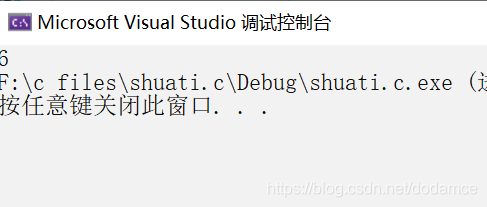3，^ 按位异或操作符

``````#include <stdio.h>
int main()
{
int a = 6;
int b = 4;
int c = a ^ b;
printf("%d",c);
return 0;
}
``````

a的二进制位 0 1 1 0
b的二进制位 0 1 0 0
-----------a^b 0 0 1 0

1，&& 逻辑与操作符

（注意与&区分，&&不是在二进制上来考虑的，只关心真假）

``````#include <stdio.h>
int main()
{
int a = 6;
int b = 4;
int c = a &&b;
printf("%d",c);
return 0;
}
``````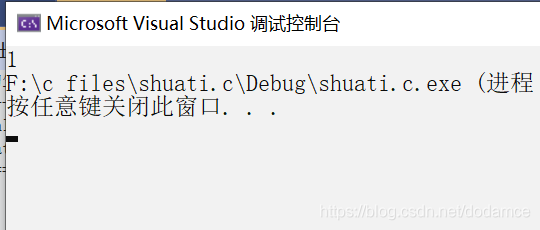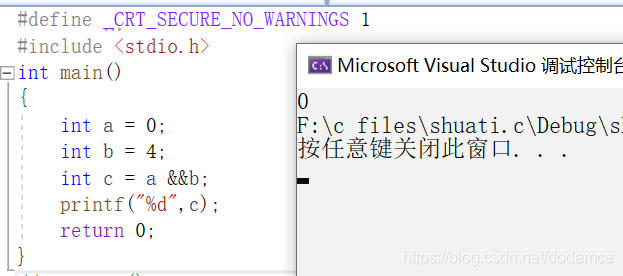注意逻辑与操作可能会出现短路现象）

``````#include <stdio.h>
int main()
{
int i = 0, a = 0, b = 2, c = 3, d = 4;
i = a++ && ++b && d++;
printf("a=%d b=%d c=%d d=%d",a,b,c,d);
return 0;
}
``````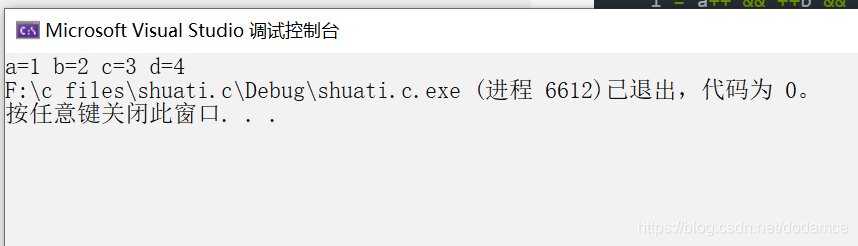分析：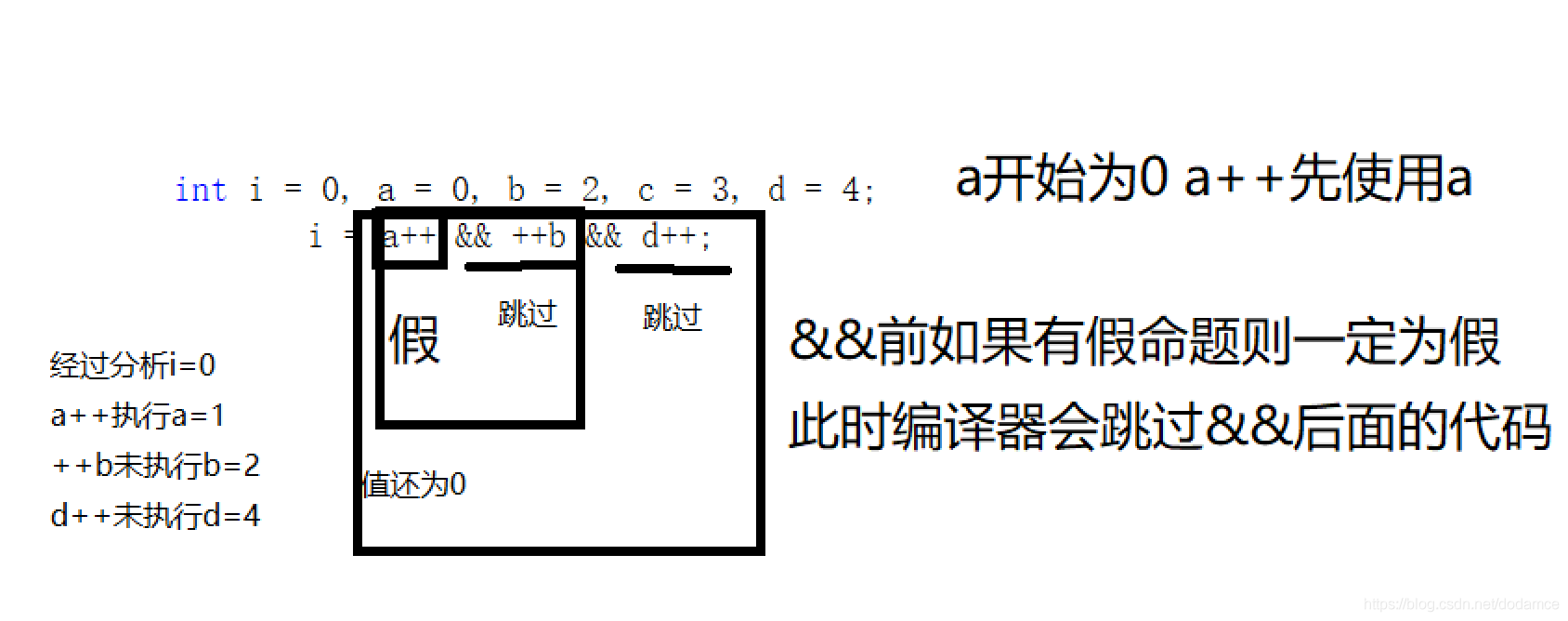``````#include <stdio.h>
int main()
{
int i = 0, a = 1, b = 2, c = 3, d = 4;
i = a++ && ++b && d++;
printf("a=%d b=%d c=%d d=%d",a,b,c,d);
return 0;
}
``````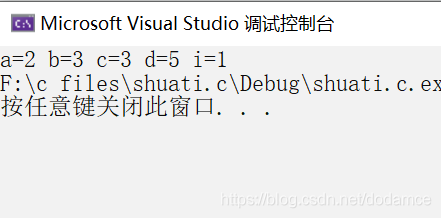2，| | 逻辑或运算符

（同理也要和 | 区分）

``````#include <stdio.h>
int main()
{
int a = 0;
int b = 3;
printf("%d",a||b);
return 0;
}
``````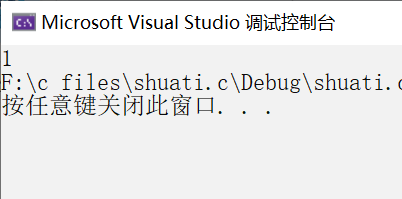``````int main()
{
int i = 0, a = 1, b = 2, c = 3, d = 4;
i = a++||++b || d++;
printf("a=%d b=%d c=%d d=%d i=%d",a,b,c,d,i);
return 0;
}
``````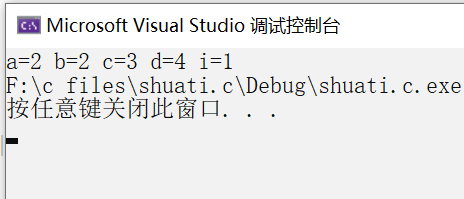if(a>2||a<0)表示a>2或者a<0

1，？： 条件操作符

eg:求三个数最大值

``````#include <stdio.h>
int main()
{
int a = 0, b = 0, c = 0;
scanf("%d%d%d", &a, &b, &c);
int max = a > b ? a : b;//先比较a,b成立max=a不成立max=b;
int max2 = max > c ? max : c;//在于c比较大于c则max是最大值，否则c是最大值
printf("最大值是%d",max2);
return 0;
}
``````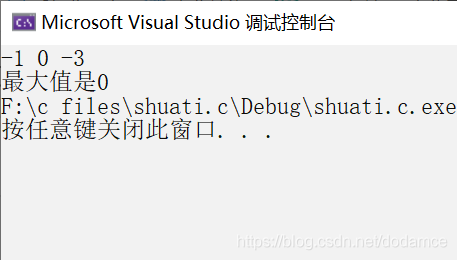1,=
C语言中=是赋值操作，不在赘述

2，a+=1——a=a+1

1， ， 逗号运算符（顺序求值操作符）

（括号内的表达式都会计算）

``````#include <stdio.h>
int main()
{
int a = 1;
int b = 2;
int c = (a > b, a = b + 10, a, b = a + 1);
printf("%d",c);
return 0;
}
``````

a=12 c=b=a+1=13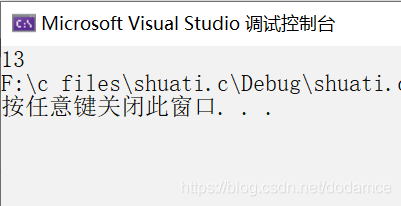应用：使代码简洁

``````while(a>0)                 while(a=fun(),a>0)
{                          {
表达式1；    可以写成        表达式1；
表达式2；                   表达式2；
a=fun();                  }
}
``````帖子
视频
声望
粉丝
社区精华内容